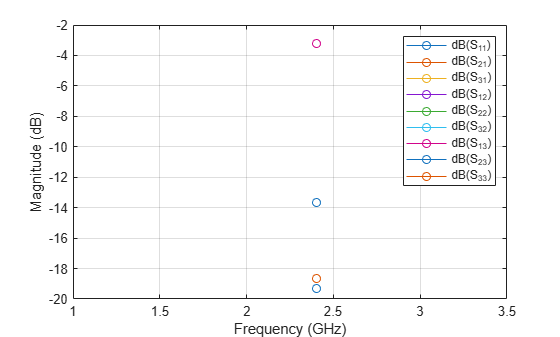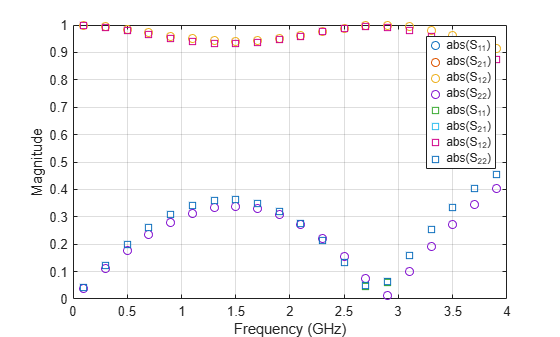# sparameters

Calculate S-parameters for RF PCB objects

## Syntax

``sobj = sparameters(rfpcbobj,freq)``
``sobj = sparameters(___,Z0)``
``sobj = sparameters(___,Name=Value)``
``sobj = sparameters(data,freq)``
``sobj = sparameters(data,freq,Z0)``
``sobj = sparameters(filename)``

## Description

````sobj = sparameters(rfpcbobj,freq)` calculates the S-parameters for the RF PCB object `rfpcbobj` over the specified frequency values.```

example

````sobj = sparameters(___,Z0)` calculates the S-parameters for the reference impedance `Z0`.```

example

````sobj = sparameters(___,Name=Value)` calculates S-parameters using one or more name-value arguments in addition to any of the input argument combinations in previous syntaxes```
````sobj = sparameters(data,freq)` creates an S-parameter object from the S-parameter data provided in `data` over the specified frequencies values.```
````sobj = sparameters(data,freq,Z0)` creates an S-parameter object for the reference impedance `Z0`. ```
````sobj = sparameters(filename)` creates an S-parameter object from the data provided in the Touchstone file specified in `filename`.```

## Examples

collapse all

Create a Wilkinson power splitter object.

`rfpcbobj = wilkinsonSplitter;`

Calculate the S-parameters for the Wilkinson power splitter at 2.4 GHz with the reference impedance of 50 ohms.

`Sobj = sparameters(wilkinsonSplitter,2.4e9,50);`

Plot the S-parameters using the `rfplot` function.

`rfplot(Sobj)`Design a microstrip transmission line at 3 GHz with 75 ohms impedance.

`m = microstripLine(Length=0.0379,Width=0.0027,Height=0.0016,GroundPlaneWidth=0.0133);`

Create a right angle bend with length equal to half the length of the transmission line and width equal to the width of the transmission line.

```layer2d = bendRightAngle( Length=[m.Length/2 m.Length/2],... Width=[m.Width m.Width]);```

Convert the right angle bend to a 3-D component.

`robj = pcbComponent(layer2d);`

Add thickness and substrate layers to the board.

```robj.BoardThickness = m.Substrate.Thickness; robj.Layers{2} = m.Substrate;```

Define frequency points to calculate the S-parameters.

`freq = (1:2:40)*100e6;`

Calculate the S-parameters of the right angle bend using the behavioral model.

`Sckt1 = sparameters(robj,freq,Behavioral=true);`

Calculate the S-parameters of the right angle bend using the electromagnetic solver.

`Sem1 = sparameters(robj,freq);`

Plot the S-parameter data using the `rfplot` function.

```rfplot(Sckt1,'abs','o') hold on rfplot(Sem1,'abs','s')```## Input Arguments

collapse all

Input object, specified as a RF PCB object. You can specify either a PCB component, a microstrip bend, or a trace. For complete list of PCB components, microstrip bends, and traces, see PCB Components Catalog and Custom Geometry and PCB Fabrication.

S-parameter data, specified as an array of complex numbers of the size N-by-N-by-K, where K represents number of frequency points.

S-parameter frequencies, specified as a scalar or vector of positive real numbers in the ascending order.

Reference impedance in ohms, specified as a positive real scalar.

Name of the Touchstone file containing network parameter data, specified as a character vector or string scalar. If the file is in the current folder or in a folder on the MATLAB® path, specify the file name. If the file is not in the current folder or in a folder on the MATLAB path, then specify the full or relative path name.

Example: `sobj = sparameters('defaultbandpass.s2p');`

### Name-Value Arguments

Specify optional pairs of arguments as `Name1=Value1,...,NameN=ValueN`, where `Name` is the argument name and `Value` is the corresponding value. Name-value arguments must appear after other arguments, but the order of the pairs does not matter.

Before R2021a, use commas to separate each name and value, and enclose `Name` in quotes.

Example: `Sobj = sparameters(robj,freq,Behavioral=true)`

Behavioral model of an RF PCB component and bend, specified as `true` (`1`) or `false` (`0`). You can compute the behavioral model for these `rfpcb` objects:

 Bends Traces Transmission line objects Inductor `spiralInductor` Capacitor `interdigitalCapacitor`

Note

• Before using the `sparameters` function to calculate S-parameters for bends and traces, convert bends and traces to PCB components using the `pcbComponent` function.

• The `sparameters` function does not support using the behavioral model argument for:

• Objects with unequal widths like `bendRightAngle`, `bendCurved`, and `bendMitered`

• Asymmetric tee and cross-junction traces

Example: ```Sobj = sparameters(microstripline,freq,Behavioral = true)```

## Output Arguments

collapse all

S-parameters, returned as an object with the following properties:

• `NumPorts` — Number of ports, N, returned as an integer. The function calculates this value automatically when you create the object.

• `Frequencies` — S-parameter frequency, returned as a scalar or row vector of length, K, in the ascending order. The function sets this property from the `filename` or `freq` input arguments.

• `Parameters` — S-parameters, returned as an N-by-N-by-K array of complex numbers. The function sets this property from the `filename` or `data` input arguments.

• `Impedance` — Reference impedance in ohms, returned as a positive real scalar. The function sets this property from the `filename` or `Z0` input arguments. If you do not provide reference impedance,, the function uses a default value of `50`.

## Version History

Introduced in R2021b